# Potential Energy for dipole in Electric Field

## Homework Statement

To derive Potential Energy for dipole p in Electric Field E.

2. Homework Equations

Potential Energy is the work done by the external agent in turning the angle of the dipole from the U=0 position to another position against the influence of the electric field applied right?

## The Attempt at a Solution

So if Torque exerted by field for a particular θ is given by $$\tau = pE\sin\theta$$
then when working out potential energy, should we not take the following:
τapp will act in same sense as dθ and opposite sense of τfield right?
So $$\tau_{app} = - pE\sin\theta$$
And the potential energy is just
$$U = \int_{\theta_1}^{\theta_2}\tau_{app}\,d\theta$$
$$U = \int_{\theta_1}^{\theta_2}-pE\sin\theta\, d\theta$$
$$U=-pE(-\cos(\theta_2)+\cos(\theta_1))$$
Now if θ1 =π/2 and θ2
$$U=pE\cos(\theta)$$
$$U=p\cdot E$$
But the traditional derivation outputs $$-p\cdot E$$ and takes τfield and not τapp in the first step. Why is this the case?

ehild
Homework Helper

## Homework Statement

To derive Potential Energy for dipole p in Electric Field E.

2. Homework Equations

Potential Energy is the work done by the external agent in turning the angle of the dipole from the U=0 position to another position against the influence of the electric field applied right?

## The Attempt at a Solution

So if Torque exerted by field for a particular θ is given by $$\tau = pE\sin\theta$$
then when working out potential energy, should we not take the following:
τapp will act in same sense as dθ and opposite sense of τfield right?
So $$\tau_{app} = - pE\sin\theta$$
And the potential energy is just
$$U = \int_{\theta_1}^{\theta_2}\tau_{app}\,d\theta$$
$$U = \int_{\theta_1}^{\theta_2}-pE\sin\theta\, d\theta$$
$$U=-pE(-\cos(\theta_2)+\cos(\theta_1))$$
Now if θ1 =π/2 and θ2
$$U=pE\cos(\theta)$$
$$U=p\cdot E$$
But the traditional derivation outputs $$-p\cdot E$$ and takes τfield and not τapp in the first step. Why is this the case?
The sign error is caused by the controversial signs of the angle and torque.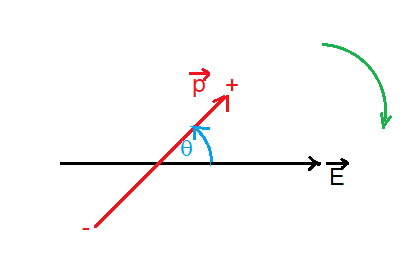You see that the torque of the electric field causes decreasing angle, clockwise (negative) direction of rotation of the dipole. So τ(field) = -p x E.

The sign error is caused by the controversial signs of the angle and torque.
View attachment 101364

You see that the torque of the electric field causes decreasing angle, clockwise (negative) direction of rotation of the dipole. So τ(field) = -p x E.

But isnt it only in the work integral that it becomes a - wrt +dθ? because Wfield=∫τfielddθcos π?

ehild
Homework Helper
But isnt it only in the work integral that it becomes a - wrt +dθ? because Wfield=∫τfielddθcos π?
What do you mean?
The electric field tends to align the dipole, decreasing the angle θ. The "natural" processes tend to make the potential energy minimum. If we set the zero of the potential energy at angle θ=pi/2, the potential energy is negative at θ=0.

What do you mean?
The electric field tends to align the dipole, decreasing the angle θ. The "natural" processes tend to make the potential energy minimum. If we set the zero of the potential energy at angle θ=pi/2, the potential energy is negative at θ=0.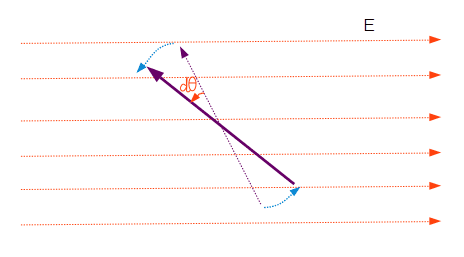τapp is -p x E will mean that it points out of the plane in this figure right? But how can that be? How do I derive potential energy in this scenario? the violet arrow is the dipole moment vector p.I know im missing something big but i am not able to figure out why..

ehild
Homework Helper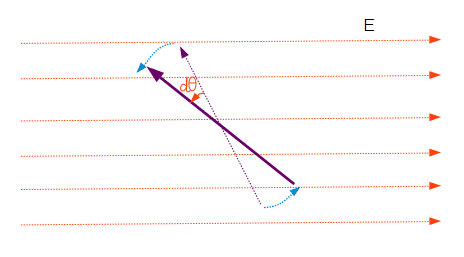τapp is -p x E will mean that it points out of the plane in this figure right? But how can that be? How do I derive potential energy in this scenario? the violet arrow is the dipole moment vector p.I know im missing something big but i am not able to figure out why..

The electric field turns the dipole in the opposite direction as in your figure, clockwise. So the torque points into the plane. Calculate the potential energy as you did in the first post, only the sign of torque was wrong.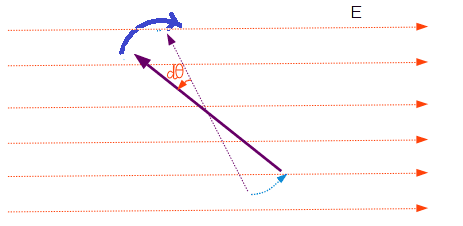The electric field turns the dipole in the opposite direction as in your figure, clockwise. So the torque points into the plane. Calculate the potential energy as you did in the first post, only the sign of torque was wrong.
View attachment 101371

Then i'd be getting the work done by the field right? Im trying to get work done by external agent in turning the dipole as in the figure in my previous post.. The turning i indicated was that produced by external torque..this work is stored in the system right?

ehild
Homework Helper
Then i'd be getting the work done by the field right? Im trying to get work done by external agent in turning the dipole as in the figure in my previous post.. The turning i indicated was that produced by external torque..this work is stored in the system right?
You need a positive external torque to turn the dipole by an angle dθ.

You need a positive external torque to turn the dipole by an angle dθ.
since τfield= p x E is intrinsically negative(?) i put the negative sign in front of it to make it positive in τapp..

ehild
Homework Helper
since τfield= p x E is intrinsically negative(?) i put the negative sign in front of it to make it positive in τapp..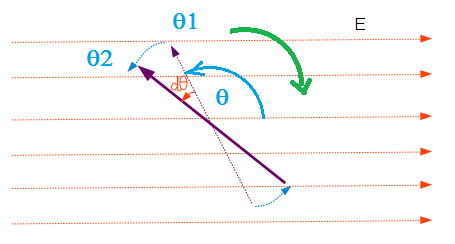The electric field tends to rotate the dipole in the direction of the green arrow, clockwise, to make it aligned with the field. To move the dipole by the red angle dθ, the external agent has to exert a torque, which would rotate the dipole in anti-clockwise direction, according to the thin blue arrows. The initial angle of the dipole with respect to the electric field is shown by the blue angle.
The elementary work of the external agent is dW=PEsin(θ)dθ, positive, as the displacement and force have the same sign. ( Both the angular displacement and the torque are positive). The work between θ1 and θ2 is equal to the potential difference U(θ2)-U(θ1) = -PE(cos(θ2)-cos(θ1)). If θ1=0 and θ2=pi/2, U(π/2)-U(0) = -PE(cos(π/2)-cos(0)). What is U(0) if U(π/2)=0?

•baldbrain
View attachment 101413
The electric field tends to rotate the dipole in the direction of the green arrow, clockwise, to make it aligned with the field. To move the dipole by the red angle dθ, the external agent has to exert a torque, which would rotate the dipole in anti-clockwise direction, according to the thin blue arrows. The initial angle of the dipole with respect to the electric field is shown by the blue angle.
The elementary work of the external agent is dW=PEsin(θ)dθ, positive, as the displacement and force have the same sign. ( Both the angular displacement and the torque are positive). The work between θ1 and θ2 is equal to the potential difference U(θ2)-U(θ1) = -PE(cos(θ2)-cos(θ1)). If θ1=0 and θ2=pi/2, U(π/2)-U(0) = -PE(cos(π/2)-cos(0)). What is U(0) if U(π/2)=0?

Ah so I have been confusing angles and magnitudes.. τapp.dθ has to be positive yes! :O
U(θ2)-U(θ1)=-pE(cos θ2-cosθ1)
so if we take U(π/2)=0, then U(0)=-pE.
Is this correct?

ehild
Homework Helper
Ah so I have been confusing angles and magnitudes.. τapp.dθ has to be positive yes! :O
U(θ2)-U(θ1)=-pE(cos θ2-cosθ1)
so if we take U(π/2)=0, then U(0)=-pE.
Is this correct?
yes.

•Curieuse
yes.
Thanks very much sir! :D

ehild
Homework Helper
You are welcome.So $$\tau_{app} = - pE\sin\theta$$
And the potential energy is just
$$U = \int_{\theta_1}^{\theta_2}\tau_{app}\,d\theta$$
$$U = \int_{\theta_1}^{\theta_2}-pE\sin\theta\, d\theta$$

This is where you are getting it wrong .

The confusion you are having is that even though $$\vec{\tau}_{app} = - \vec{p} \times \vec{E}$$ , $$W_{app} = U = \int (pEsin\theta) d\theta$$ .

So τ(field) = -p x E.

There should be no minus sign . Except this , I agree with everything you have written.

•Curieuse
ehild
Homework Helper
There should be no minus sign . Except this , I agree with everything you have written.
Yes, τ(field) = p x E, but with the angle shown, it is -pEsin(θ).
The vector product uses the sin of the angle the second vector encloses with the first, anti-clockwise. In this problem, we used the angle the first vector (p) made with second (E). In this way, the field produced negative torque.

Yes, τ(field) = p x E, but with the angle shown, it is -pEsin(θ).

If by putting a minus sign you are representing a clockwise torque ,then it is alright . But if the - sign has something to do with the way angle is measured , then I do not understand .

The vector product uses the sin of the angle the second vector encloses with the first, anti-clockwise.Why complicate things ?

The cross product uses the smaller of the two angles between the vectors when joined from tail to tail .

ehild
Homework HelperWhy complicate things ?

The cross product uses the smaller of the two angles between the vectors when joined from tail to tail .
Yes, calculating the magnitude of the torque. But from τ = pxE, it does not follow that τ=pEsinθ, where 0 ≤ θ ≤ π. The torque has sign, in addition that its direction is perpendicular to both p and E, which has to be taken into account when calculating work.

Last edited:
The torque has sign, in addition that its direction is perpendicular to both p and E, which has to be taken into account when calculating work.

I believe that the sign of torque is not required for calculating work because work ( dot product ) requires magnitude of torque . All that matters is that whether the torque tries to increase or decrease the angle . When calculating work , the minus sign is placed when torque tries to decrease the angle between the vectors .

ehild
Homework Helper
I believe that the sign of torque is not required for calculating work because work ( dot product ) requires magnitude of torque . All that matters is that whether the torque tries to increase or decrease the angle . When calculating work , the minus sign is placed when torque tries to decrease the angle between the vectors .
Trying to increase or decrease angle is connected to the direction of torque.

•conscience